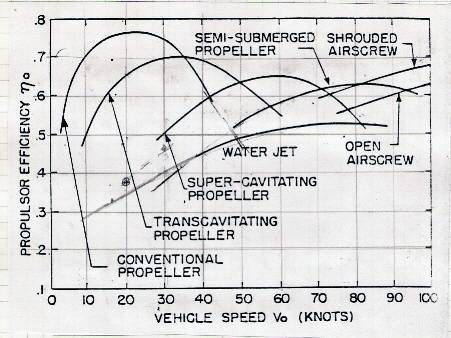# Speed as a function of time

How could I express the speed of an object as a function of time if the object is accelerating with a constant power output and is being affected by quadratic (Rayleigh) drag?

SteamKing
Staff Emeritus
Homework Helper
What type of object?

Well, it's supposed to be a submarine.
As far as the physics go, it's a solid object with density equal to the density of the liquid
Anything else?

SteamKing
Staff Emeritus
Homework Helper
As a start, I would draw a free-body diagram and add the relevant forces. Since it is a submarine, the power produced by the propulsion machinery is converted into thrust, either by turning a propeller or by means more exotic. The drag is proportional to the velocity squared and acts to oppose the thrust. Since the sub is accelerating, then T - D is positive, and T - D = m a, where m is the mass of the submarine, and a = dv/dt.

A.T.
Since it is a submarine, the power produced by the propulsion machinery is converted into thrust, either by turning a propeller or by means more exotic.
And in general the thurst will not be constant for constant power input, but depend on the speed.

And in general the thurst will not be constant for constant power input, but depend on the speed.

And that's the point where I got stuck. I found an equation that works if the thrust is constant but that is not the case here.

Perhaps if I expressed drag as a loss in energy (speed3) it might be simpler.

Not quite sure though, we haven't done anything like this at school so far and it might be a few years till we do.

A.T.
And that's the point where I got stuck. I found an equation that works if the thrust is constant but that is not the case here.
You won't get an analytic solution for this. The thrust itself is not some simple function but usually derived empirically or numerically. You have a chart like this:And get the thrust by:

thrust = (efficiency * shaft_power) / velocity

To get the speed as function of time you have to integrate the acceleration from the net force (thrust - drag) numerically. For low velocities there is static thrust data.

Last edited: